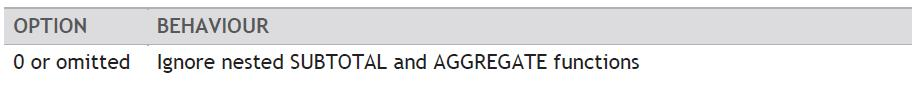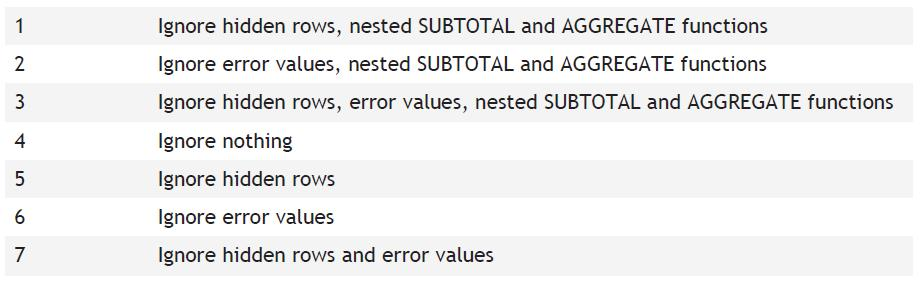# Business Automation and Software Blog

Excel Tips and Tricks / Business Reporting Question: How do I calculate the sum for a range of numbers with error values within the range? Using the standard sum function in Microsoft Excel returns an error.

Answer: By using the Aggregate function in MS Excel 2010.

Why: To find the sum for a range of numbers where there are error values within the range.

Description: The AGGREGATE function addresses the limitation of conditional formatting. Data bars, Icon Sets and Color Scales cannot display conditional formatting if there are errors in the range. This is because the MIN, MAX and PERCENTILE functions do not calculate when there is an error in the calculation range. The LARGE, SMALL, and STDEVP functions also affect the appropriate functionality of certain conditional formatting rules for the same reasons. By using the AGGREGATE function, you can implement those functions because the errors will be ignored. In addition, the AGGREGATE function can apply different aggregate functions to a list or database with the option to ignore hidden rows and error values.

Syntax: AGGREGATE(function_num, options, ref1, [ref2], …)

Applies To: Excel 2010

1. Refer to the data given below: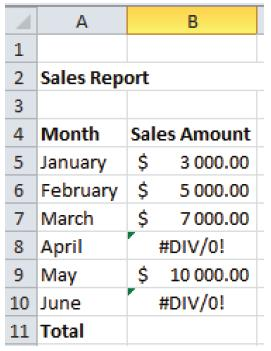2. Select B11 and enter; =sum(B5:B10) and press enter.
3. An error message will be displayed in the Total. Delete the error message in cell B11.
4. To overcome this limitation, we shall use the Aggregate function.
5. Select cell B11.
6. Select Formulas, Insert Function and search for the Aggregate function.
7. Select OK.
8. Select as below: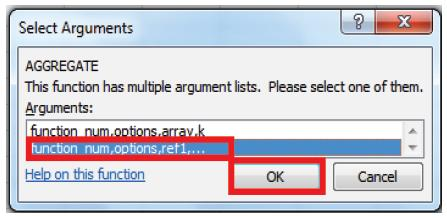9. Enter as below: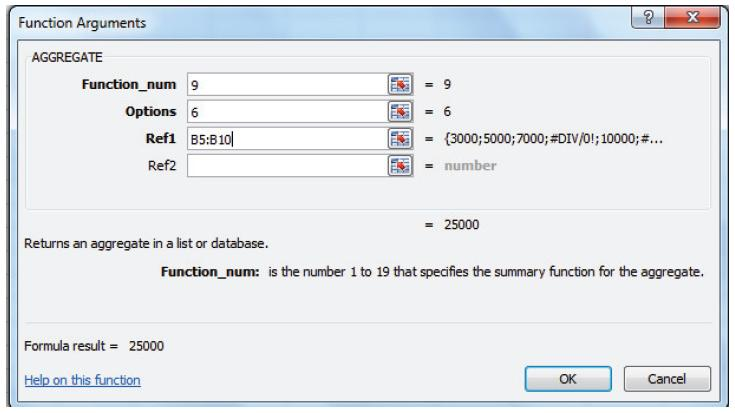10. Select OK.

11. The answer will be as below: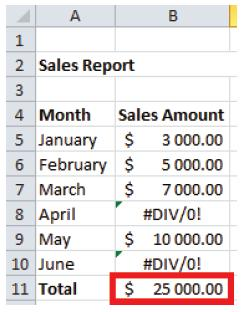The following numbers represent some function numbers than can be used in the formula above:

1=Average,2=Count,3=CountA,4=Max,5=Min,9=Sum.

For Option numbers refer to the table below.